# Geometric figures

We can find them practically everywhere: on the wheels of cars (circles), on a piece of pizza (triangle), in buildings (rectangles), on umbrellas (octagon), in the eyes of a friend (ellipse), on some roofs of houses (pyramids), among others. A geometric figure it is a closed space limited by points, lines or surfaces. Those that are two-dimensional are called polygons, those that are solid or three-dimensional, polyhedra.

## Definition

In general, a geometric figure is a non-empty set whose elements are points.

### Polygons

Many of the polygons (two-dimensional geometric figures) can be defined as a set of points (or vertices) and lines connecting the points of a closed chain, as well as the resulting interior points, among them are triangles, squares and pentagons. Others may be bounded by curves, such as the circle or the ellipse.

### Polyhedra

On the other hand, many of the polyhedra (three-dimensional geometric figures) can be defined by a set of vertices, lines that connect to these and the two-dimensional faces delimited by lines, as well as the resulting interior points. These include cubes, pyramids, and tetrahedrons. Others may be limited by curved surfaces, such as the ellipsoid and the sphere.

## List of some trigonometric figures

### Dimension 0 (dimensionless)

• Point: it is a geometric figure without dimension, it does not have length, area, volume, or any other dimensional angle. It is not a physical object.

### Dimension 1 (linear)

• Segment: is a fragment of a line.• Curve: it is a continuous line of one dimension, which changes direction gradually. Represents some mathematical function.

### Dimension 2 (superficial)

• Circle: it is a flat surface bounded by a curved line (circumference).• Triangle: is a three-sided polygon.• Quadrilateral: is a polygon that has four sides. There are two types: convex and concave.

The convex quadrilaterals are those that when taking two interior points A and B from it, all the points of segment AB are inside the quadrilateral.The concave (or non-convex) quadrilaterals are those where two interior points A and B of the same can be found, such that some of the points of segment AB are outside the quadrilateral.• Parallelogram: is a convex quadrilateral whose pairs of opposite sides are equal and parallel.

The square: has all sides of equal length.The rectangle: has its opposite sides of equal length.The rhombus: it has all its sides of equal length, and two pairs of equal angles.The rhomboid: has opposite sides of equal length and two pairs of equal anglesTrapeze: it is a quadrilateral that has two parallel non-consecutive sides. There are several types (rectangle, isosceles, scalene).### Three dimensions (volumetric)

• Cube: is a congruent square six-sided polyhedron.• Cylinder: It is a solid or hollow figure with a circular or oval base and whose straight sides are parallel.• Cone: is a solid or hollow figure that tapers from a circular base to a point outside it.• Wait: It is a closed solid figure delimited by a surface in which all the points are equidistant from a central point called the center.• Pyramid it is a polyhedron that has a polygonal face and the other faces are triangles with a common vertex. The polygon is the base of the pyramid. The triangles are the side faces. The common vertex of these lateral faces is the vertex of the pyramid.• Prism: It is a polyhedron with two congruent polygons, parallel, as bases, while all the other faces are parallelograms.## Areas of some superficial geometric figures

 Circle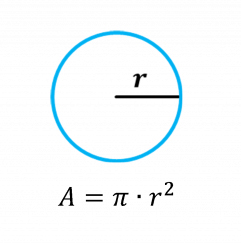Triangle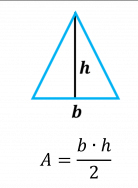Square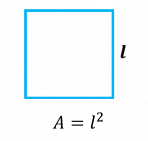Rectangle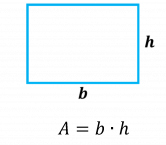Diamond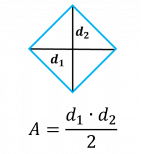Trapeze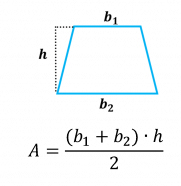Parallelogram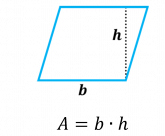## Areas and volume of some geometric volumetric figures

 Cube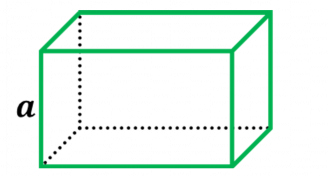Cylinder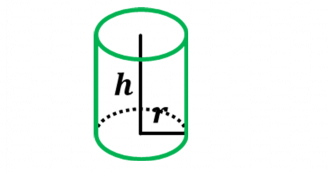Cone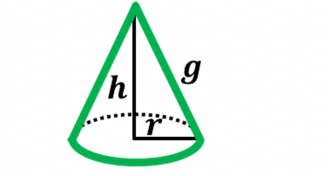Sphere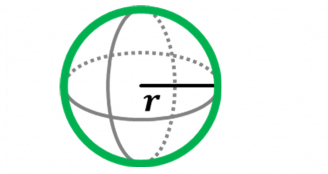Pyramidwhere:PB = perimeter of the baseAB = Base area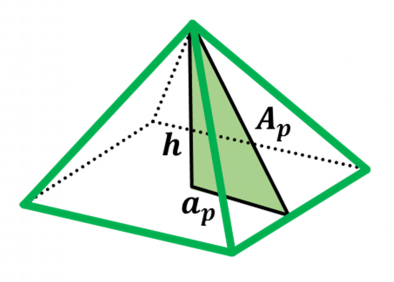AL = lateral area; AT = Total area; V = Volume